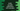# Kotlin groupBy method explanation with example## Kotlin groupBy introduction :

groupBy method of Kotlin is used to group similar items of a collection. It is useful in many cases like we can group items based on type, length, object category, etc. It takes one selector function and based on that function it groups the items.

The return value is a map with keys and lists of groups. For example, if we use a character array, it will return the groups of characters. In this tutorial, we will learn how to use Kotlin groupBy with different examples.

### Syntax of groupBy :

Below is the syntax of groupBy method :

``````inline fun <T, K> Array<out T>.groupBy(
keySelector: (T) -> K
): Map<K, List<T>>``````

Here, keySelector is the selector function used to group the items.

### Example of groupBy: Group strings of equal length :

Let me show you one quick example of how to use groupBy. The below program will group the array items based on their lengths :

``````fun main() {
val strArray = arrayOf("one", "two", "three", "four", "five", "six")

val strMap = strArray.groupBy { it.length }

print(strMap)
}
``````

It will print the below output :

``````{3=[one, two, six], 5=[three], 4=[four, five]}
``````

Similarly the below example will group the strings by the first character :

``````fun main() {
val strArray = arrayOf("one", "two", "three", "four", "five", "six", "seven", "eight", "nine", "ten")

val strMap = strArray.groupBy { it.first() }

print(strMap)
}
``````

It will print :

``````{o=[one], t=[two, three, ten], f=[four, five], s=[six, seven], e=[eight], n=[nine]}
``````

It maintains the order while producing the keys. For example, if we change the above array to :

``````val strArray = arrayOf("ten", "nine", "eight", "seven", "six", "five", "four", "three", "two", "one")
``````

It will result the below output :

``````{t=[ten, three, two], n=[nine], e=[eight], s=[seven, six], f=[five, four], o=[one]}
``````

### Using groupBy with a transform function :

We can also pass one value transform function as the second argument of groupBy. This function is applied to each value before the first function is called. For example :

``````fun main() {
val strArray = arrayOf("one", "two", "three", "four", "five", "six", "seven", "eight", "nine", "ten")

val strMap = strArray.groupBy ({ it.first()}, {it.toUpperCase()})

print(strMap)
}
``````

It will give the below output :

``````{o=[ONE], t=[TWO, THREE, TEN], f=[FOUR, FIVE], s=[SIX, SEVEN], e=[EIGHT], n=[NINE]}
``````

### Using multiple keys in groupBy :

Multiple keys are used to check multiple conditions while grouping. For custom objects, it is useful. For example, we can group by age and the first character of the name of an employee. We need to create one data class for that. For example :

``````data class Employee(val age: Int,val name: String)
data class EmployeeGroup(val firstChar: Char, val age: Int)

fun Employee.getKey() = EmployeeGroup(this.name.first(), this.age)

fun main() {
val strArray = arrayOf(Employee(25,"Alex"), Employee(25,"Bob"), Employee(30, "Albert"),
Employee(30,"Bella"))

val strMap = strArray.groupBy ({ it.getKey()})

print(strMap)
}
``````

Here, we are grouping the objects of Employee based on the first character of the name and age. EmployeeGroup is a data class with one character and an integer. It will give the below output :

``````{EmployeeGroup(firstChar=A, age=25)=[Employee(age=25, name=Alex)], EmployeeGroup(firstChar=B, age=25)=[Employee(age=25, name=Bob)], EmployeeGroup(firstChar=A, age=30)=[Employee(age=30, name=Albert)], EmployeeGroup(firstChar=B, age=30)=[Employee(age=30, name=Bella)]}
``````